# Naive Bayes Algorithm in Machine Learning

## Naive Bayes Algorithm in Machine Learning

In this article, I am going to discuss the Naive Bayes Algorithm in Machine Learning with Examples. Please read our previous article where we discussed the Recommendation Engine and its Working in Machine Learning with Examples.

##### Naive Bayes Algorithm in Machine Learning

A probabilistic machine learning model called a Naive Bayes classifier is utilized to perform classification tasks. The Bayes theorem lies at the heart of the classifier.

##### Let’s understand what is Bayes Theorem first.

The Bayes’ Theorem calculates the chance of an event occurring given the probability of a previous event. Mathematically it can be expressed as –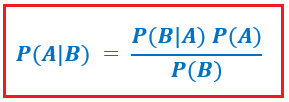P(B) 0, where A and B are events.

• Essentially, we’re looking for the likelihood of event A if event B is true.
• The prior of A is P(A) (the prior probability, i.e. Probability of event before evidence is seen). The evidence is a value assigned to an unknown instance’s attribute (here, it is event B).
• P(A|B) is the a posteriori probability of B, that is, the likelihood of an event after it has been observed.
• The evidence is B, and the hypothesis is A.
##### The basic Naive Bayes assumption is that each feature contributes to the outcome in an equal and independent way.

The predictors/features are assumed to be independent in this case. That is, the presence of one attribute has no bearing on the other, which is impossible in the case of real-world data sets. As a result, it is said to as naïve.

We can rewrite the above in terms of x and y where, x is the independent feature while y represents a dependent feature, whose possibility of occurrence needs to be calculated given x has already occurred.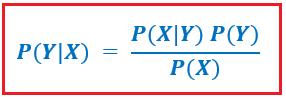Let’s consider an example of a dataset with n independent features (. Now assume we have to calculate the probability of event Y occurring given X. Let’s substitute these values for x and expand using the chain rule. Hence, the result will be –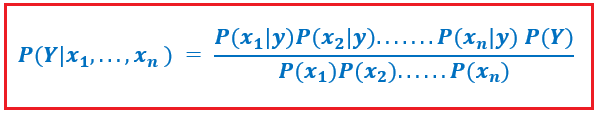You can now seek up the values for each from the dataset and plug them into the equation. The denominator does not change for any of the entries in the dataset; it remains constant. As a result, the denominator can be eliminated and proportionality introduced.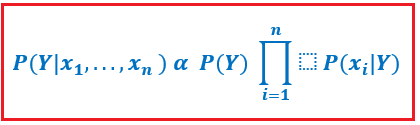The class variable(y) can have two outcomes and multivariate in some circumstances as well. As a result, we must find the class y with the highest probability.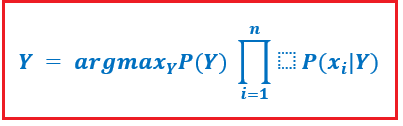Let’s understand all of this through an example. Consider a hypothetical dataset describing the meteorological conditions for a game of badminton. Each tuple assigns a fit(“Yes”) or unfit(“No”) rating to the weather circumstances when playing badminton.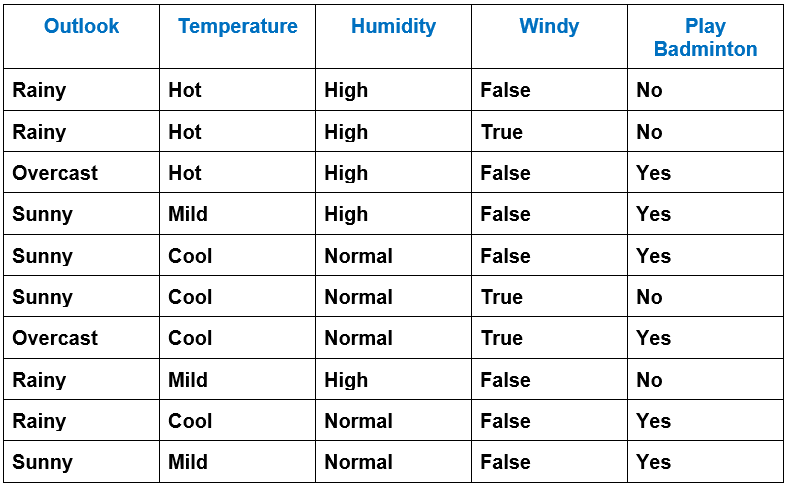Let’s try manually applying the following formula to our weather collection. We’ll need to make some precomputations on our dataset to do this. For each in X and in y, we must calculate P( | ). The following tables demonstrate all of these calculations:

##### Outlook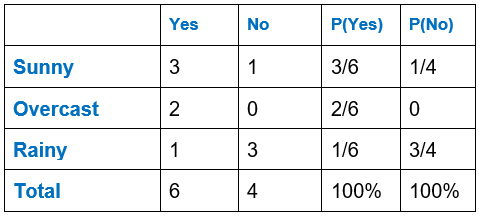##### Temperature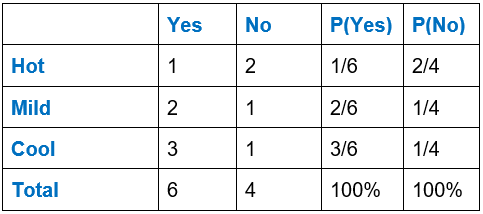##### Humidity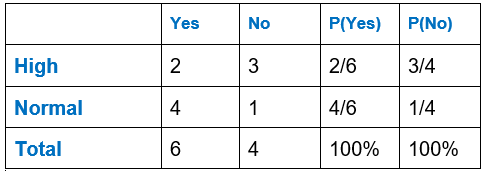##### Wind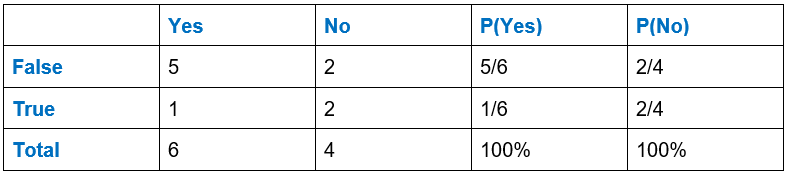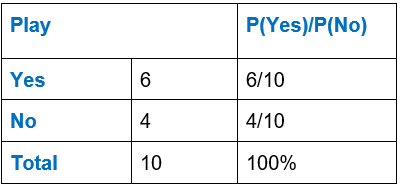In the next article, I am going to discuss SVMs in Machine Learning with Examples. Here, in this article, I try to explain the Naive Bayes Algorithm in Machine Learning with Examples. I hope you enjoy this Naive Bayes Algorithm in Machine Learning with Examples article.